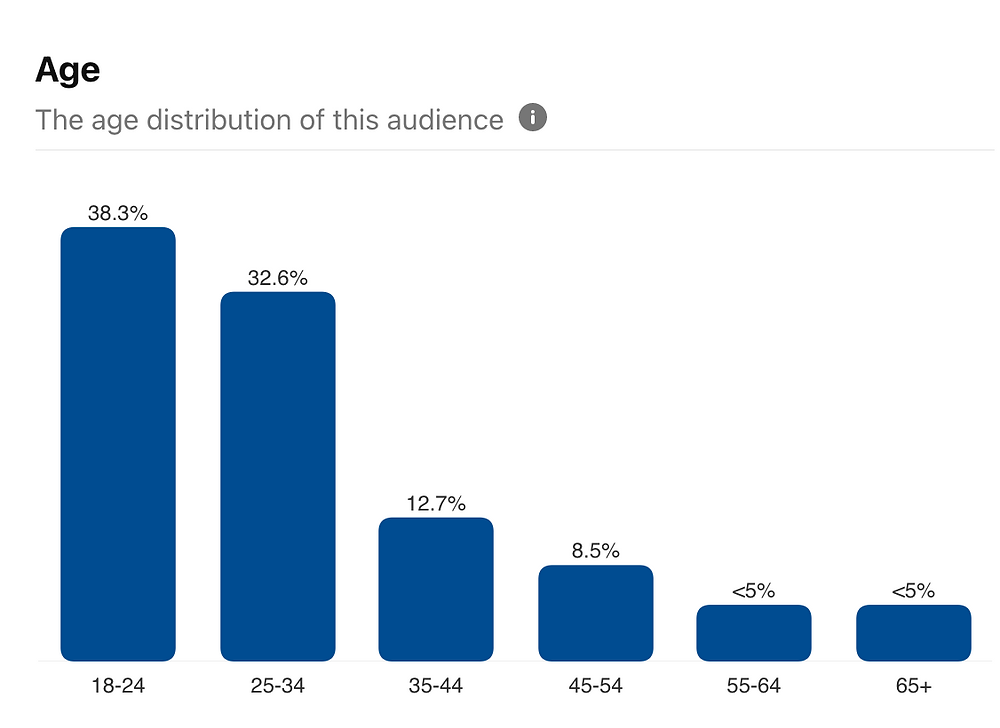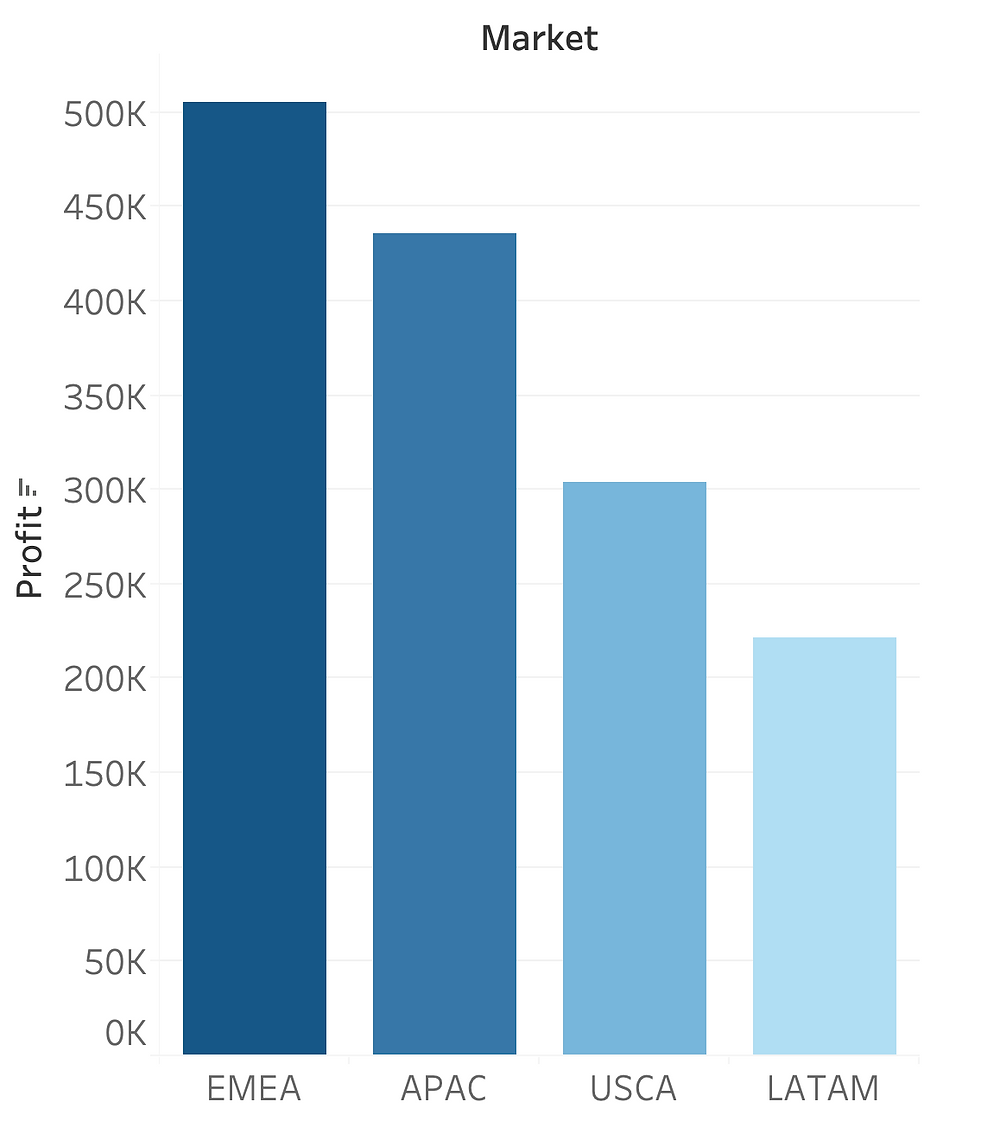Featured Articles

Search

# How to Choose the Most Appropriate Chart?

Updated: Nov 21, 2020In this information rich age, data visualizations are designed to make the knowledge transfer between deliverers and receivers easier. Therefore, it is crucial for the dashboard creators to know which chart is aligned with the key delivery objectives. On the other hand, having a basic understanding of the underlying meaning of each chart also helps the audience to interpret dashboards effectively. In this article, I introduced a way that may help to better understand some common charts and graphs, e.g. scatter plot, map, pie graph and stacked bar chart etc, by categorising them into four main types: distribution, comparison, composition and correlation. It doesn't mean that it is a clear-cut solution or a rigid boundary that limits us to only use a chart in one certain way. Rather, it is a conclusion drawn from my experience regarding what is the main objective each chart is able to communicate. Moreover, designing effective dashboards is beyond choosing appropriate charts. Read more dashboard design principles in this article.

## 1. Distribution

This type of data visualization helps to interpret univariate analysis result in the early analytical stage. Simply put, it shows where data points are dense and where they are sparse in one dimension. Distribution charts can also be widely applied in market research, such as demographics analysis and customer segmentation. Some of the common charts under this category are histogram, box plot and map. However, I am more leaning towards categorizing box plot into "comparison" type, which I will explain it in the later section.

### Histogram

Histogram looks very similar to bar chart because, oh well, it is also composed of bars. However, instead of comparing the categorical data, it breaks down a numeric data into interval groups and shows the frequency of data fall into each group. It is commonly used to gain insights about your customers, e.g. Pinterest use histograms to show the age distribution of your audience. Histogram is good at identifying the pattern of data distribution on a numeric spectrum. For example, it magnifies what is the most probable value range and whether the data is skewed or centred.Pinterest audience analytics

### Map

Map is also frequently used to show demographical data. By linking to the geospatial data, it indicates where are your audience or customers located. The logic behind map charts is that numeric values are aggregated by a geospatial attribute (e.g. regions, city, country or state etc). Then use gradient colors to represent the variations in data density among locations. In the graph below, regions with higher values are in darker color and vice versa.Map

## 2. Comparison

It is hard to compare which number is larger or smaller when we are displayed in a table or spreadsheet. Adding a visual element to the comparison and contrast significantly reduces the amount of time and mental energy required to interpret the data. These visual representations can be achieved through bar chart, line chart or box plot.

### Bar Chart

Bar chart compares the measure of categorical dimension. As we can see, comparing the height of each bar gives us a more intuitive perception than looking at the table alone. Bar chart is very similar to a histogram. The fundamental difference is that the x-axis of bar charts is categorical attribute instead of numeric interval in the histogram. For example, in this chart, we compare the profit value of each market "EMEA", "APAC" ... Whereas in a histogram, we break down a numeric attribute age into intervals "18-24", "25-34" ...represent data in table formatrepresent data in bar chart

Furthermore, bar chart is not just limited to plot one categorical data. An extension of bar chart, clustered bar chart (or group bar chart) compares two categorical attributes. For example, the comparison of market profit can be further broken down into different year segments. This allows us to compares based on market to market and also based on different periods of order time.clustered bar chart

### Line Chart

It indicates trends and developments of numeric data over time. It is commonly used in time series analysis, by visualizing the fluctuation of a numeric variable against a date-type variable. Each line itself is a comparison between one historical time point and another. Additionally, we can introduce a categorical attribute and use distinct colors to bring out the contrast of each category. For example, the chart below plots the number of orders over time and each line indicates one category of customer segments. Therefore, horizontally it illustrates the time series analysis of order quantities. While by comparing the line vertically, we can draw out the conclusion that the number of orders differs remarkably among various segments.number of orders over time

### Box Plot

Some people may argue that box plot should be categorized as "distribution" chart, as it is mainly used for showing data distribution through percentile. It is true that looking at one box plot alone, it indicates where the are 25%, 50% and 75% percentile. Additionally, a slight twist of bringing down the opacity of each data point provides more direct visualization of the distribution.

However, box plot is rarely used alone. Instead, it is usually used to compare multiple groups of data. For example, it is a great complimentary tool for ANOVA test because it illustrates the variation across groups and within groups. Therefore, its functionality is beyond just a simple univariate analysis. In the box plot below, not only does it shows the difference of data within an individual subject group, but also it displays the variation among critical reading group, mathematics group and writing group.box plot

## 3. Composition

Data visualizations that fall under this category provide a bird-eye view. For instance, pie chart, stacked bar chart and area chart are designed to illustrate the part-to-whole relationship.

### Pie Chart

It is used to represent the percentage and weight of components belonging to one categorical attribute. The size of the pie slice is proportional to the percentage, hence it intuitively depicts how much each component occupies the whole.pie chart

### Stacked Bar Chart

Stacked bar chart is used when we need to break down a primary category into a secondary category. As we can see in the chart below, it is very similar to the bar chart we saw earlier. Horizontally, it also compares the performance of each market. Vertically, it further demonstrates the composition of each segment within the market.stacked bar chart

### Area Chart

Area chart mapped the measure of a categorical dimension against a date-type variable. The chart below shows how the profit of each product category fluctuates over time. It is different from line chart, since the measure is accumulated and stacked from bottom to top. Therefore, it can be utilized to illustrate how each category contributes to the whole throughout the timeline. It also helps to visualize how the profit composition changes over time by comparing the variation in area size.area chart

## 4. Correlation

Correlation charts assist in discovering whether one ore more pairs of variables are related. It mainly indicates a dependency between variables instead of a causal relationship (causal, NOT "casual", relationship means that variable x causes the changes in variable y). Scatter plot and heatmap are great tools to depict correlation.

### Scatter Plot

It plots one numeric attribute against another numeric attribute and visualizes the correlation between axes. Scatter plot is commonly applied to identify regression type of relationships such as linear regression, logistic regression etc. It also provides a robust analysis of the correlation significance. We can estimate that the correlation relationship is stronger when the data points are concentrated on certain areas, whereas the relationship is weak if they are sparse.scatter plot

### Heatmap

Heatmap is commonly used as a visual representation of correlation matrix. It is a powerful technique to find correlated attributes in principle component analysis (PCA). By using a gradient color code, we can directly visualizate which attributes-pairs are strongly correlated. In the heatmap below, highly positively correlated attributes are in darker blue.heatmap

## More Resources That May Help!

Dashboard Design Principle